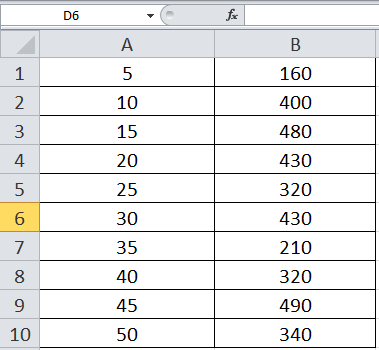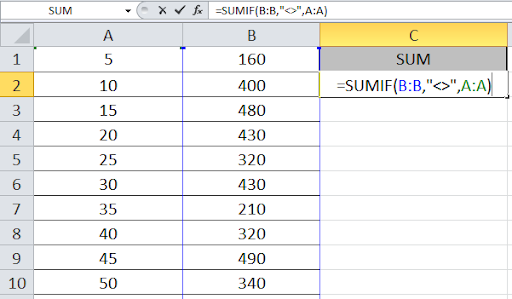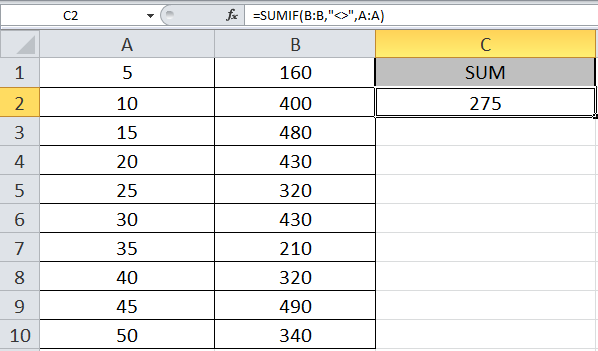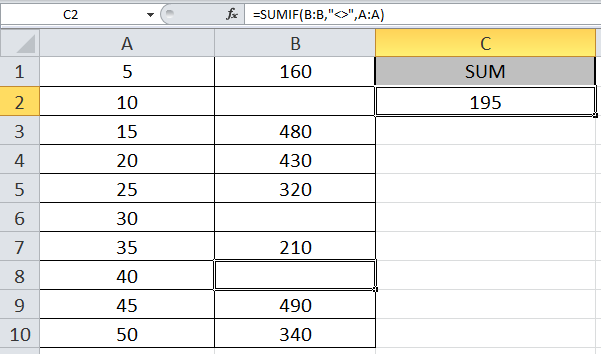# How to SUMIF with Not Blank Cells in Excel

When you need to use excel SUM if function with some condition, we use the SUMIF function in Excel.
SUMIF Excel function gets the sum of range based on the condition and returns the recorded values.
Syntax:

 =SUMIF(range, criteria , [sum_range])

Let’s understand how to do sumif in excel  with an example shown below

I want a formula preferably SUMIF function to sum the numbers in column A only if the corresponding cell in column B is not empty. I have tried to use sumif multiple columns based on non-blank cells.So write the formula where you want to get the sum

 =SUMIF(B:B,"<>",A:A)

Explanation:
The function checks the cells which are not blank and excel if the cell is not blank, it records the value of the corresponding cell. The function returns the sum of the recorded values.

Sum of values in Column A is done, a condition that the corresponding Column B should not be blank.Press Enter to get the desired result.Now we will remove some of the numbers from Column B.As you can see, as I removed some numbers, the sum changed. The Formula holds the blank cells and considers only the non-blank cells in Column B and returns the sum of corresponding values in Column A.

In this way, we learnt how to use SUM IF function in Excel to get a return from the non-blank cells. You can use these functions in Excel 2016, 2013 and 2010. Find more articles on Mathematical formulation with condition here. If you have any issue regarding this article or any unresolved query, please comment in the comment box below.

Related Articles:

How to Calculate Only If Cell is Not Blank in Excel

Adjusting a Formula to Return a Blank

Checking Whether Cells in a Range are Blank, and Counting the Blank Cells

Only Return Results from Non-Blank Cells

Popular Articles:

50 Excel Shortcut to Increase Your Productivity

How to use the VLOOKUP Function in Excel

How to use the COUNTIF function in Excel 2016

How to use the SUMIF Function in Excel

Terms and Conditions of use

The applications/code on this site are distributed as is and without warranties or liability. In no event shall the owner of the copyrights, or the authors of the applications/code be liable for any loss of profit, any problems or any damage resulting from the use or evaluation of the applications/code.